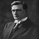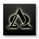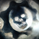3400 뷰
3400
This is a new indicator release, Using the principle of Murrey Math Line Trading Systems. It will be easier for someone to add alerts on an oscillator rather than a overlay.

Currently, I did add some aesthetics for those who like to view different colors, can be turned off.

Oscillator Color Definition -
Green = Above MidLine
Red = Below Midline
Blue = Below Negative 3rd Quadrant
Orange = Above Positive 3rd Quadrant

/////////
Planned future Improvement is to consider Wicks as well.

Do post your opinions and any improvement.

GL.
// Created by UCSgears
// Modified the conceptual Murray Math Lines System overlay to an Oscillator
// Easier to add Alerts using an oscillator rather than an overlay
// Does provide some aesthetics, Will be improved as Time Progresses

study(title="UCS_Murrey's Math Oscillator", shorttitle="UCS_MMLO", overlay=false, precision = 2)
// Inputs
length = input(100, title = "Look back Length")
mult = input(0.125, title = "Mutiplier; 1/8 = 0.125; 1/4 = 0.25.....")
lines = input(true, title= "Show Murrey Math Fractals")
bc = input(true, title = "Show Bar Colors for Oversold and Overbought")
// Donchanin Channel
hi = highest(high, length)
lo = lowest(low, length)
range = hi - lo
multiplier = (range) * mult
midline = lo + multiplier * 4

oscillator = (close - midline)/(range/2)

scolor = oscillator > 0 and oscillator < mult*6 ? green : oscillator < 0 and oscillator > -mult*6 ? red : oscillator < -mult*6 ? blue : oscillator > mult*6 ? orange : na

plot (oscillator, color = scolor, title = "Murrey Math Oscillator", style = columns, transp = 60)
plot(0, title = "Zero Line", color = gray, linewidth = 4)

plot(lines == 1 ? mult*2 : na, title = "First Positive Quadrant", color = gray, linewidth = 1)
plot(lines == 1 ? mult*4 : na, title = "Second Positive Quadrant", color = gray, linewidth = 1)
p3 = plot(lines == 1 ? mult*6 : na, title = "Third Positive Quadrant", color = gray, linewidth = 1)
p4 = plot(lines == 1 ? mult*8 : na, title = "Fourth Positive Quadrant", color = gray, linewidth = 1)
plot(lines == 1 ? -mult*2 : na, title = "First Negative Quadrant", color = gray, linewidth = 1)
plot(lines == 1 ? -mult*4 : na, title = "Second Negative Quadrant", color = gray, linewidth = 1)
p2 = plot(lines == 1 ? -mult*6 : na, title = "Third Negative Quadrant", color = gray, linewidth = 1)
p1 = plot(lines == 1 ? -mult*8 : na, title = "Fourth Negative Quadrant", color = gray, linewidth = 1)

fill (p1,p2, color = orange)
fill (p3,p4, color = lime)

// Bar Color Oversold and Overbought
bcos = bc == 1 and oscillator > mult*6 ? orange : na
bcob = bc == 1 and oscillator < -mult*6 ? blue : na

barcolor (bcos)
barcolor (bcob)

## 코멘트Great idea, USCGEARS, I'm a big MurreyMath devotee, and you may want to experiment with these look-back periods, based on T H Murrey's own suggestions, for example for Swing, or Position traders, change the look-back-period from 100 to 64, as 8x8=64, or cut that in half to LBP= 32. Shorter-term and day-traders may want to use a LBP of 16, or real aggressive scalpers try a LBP of 8!
응답Fantastic and exceeded expectations. Cannot wait to start using it.
응답You were sure to get a big thumbs up from me on the concept. Thank you. Since you asked for suggestions, I might suggest a a slightly modified color scheme to consider the movement between fractal levels rather than just the absolute quartile extemes and reserve your "cautionary colors" for falling from oversold or rising from oversold since those would be the transitional periods between the most extreme fractals. So the change would be the current yellow becomes different color of green (maybe a very bright or very dark green showing the extreme quartile has been reached) and only remains yellow if it is falling from a prior higher quartile to a lower one. Likewise the blue would be a different shade of red if intensifying downside and entering last quartile but only be blue color on the extreme oversold and moving to the next higher quartile. Thus your highlight colors would not merely show the highest quartile extremes but he transitions from the extremes which seem most likely to produce the biggest trading opportunities. Candles / bars could have correspeonding colors and/or highlight the transitional alert colors showing the potential turning regions.
응답Alphaoptions
Yes, Sure, will do that in the version 2. Just wanted to put out first and see the response, before I will continue my work on it.
응답